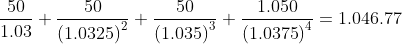Scan QR code or get instant email to install app

Question:

# Given only this information, the price of a 2-year, semiannual-pay, 10% coupon bond with a face value of $1,000 is closest to: A$1,046.77.
explanation

1212This question can be answered without calculations. Since the spot rates are less than the coupon rate, the price must be greater than par value, so C is the only possible correct choice.
This is a four-period bond with 50 cash flows each period. Divide each spot rate by two to get the semiannual rate.
PV1: N = 1; I/Y = 3.00; FV= 50; CPT->PV= 48.54
PV2: N = 2; I/Y = 3.25; FV= 50; CPT->PV= 46.90
PV3: N = 3; I/Y = 3.50; FV= 50; CPT->PV=45.10
PV4: N = 4; I/Y = 3.75; FV= 1,050; CPT->PV = 906.23
OrSum to get 1,046.77.